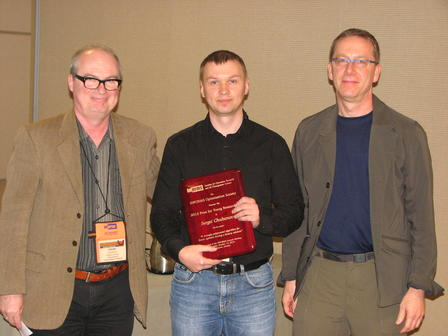# 2012

## Sergei Chubanov is the winner of the 2012 INFORMS Optimization Society Young Researcher PrizeEndre Boros (left), Sergei Chubanov, and Jon Lee.

## Citation

The 2012 recipient of Prize for Young Researchers of the INFORMS Optimization Society is Sergei Chubanov of the University of Siegen, for his paper "A strongly polynomial algorithm for linear systems having a binary solution", Mathematical Programming, Volume 134, Number 2 (2012), 533-570.
Linear programming is fundamental in mathematical optimization, is widely used, and has had major impact on numerous branches of mathematics, engineering, and the sciences. An intriguing open end in the theory of linear programming is the existence of a strongly polynomial algorithm. The cited paper provides an important contribution to this open problem. The paper considers linear programs having a feasible region within the unit cube, and provides a strongly polynomial algorithm that solves such a linear program if it has a feasible binary solution, or proves that no such binary solution exists. Let us note that many linear programs arising, e.g., in combinatorial optimization have feasible binary solutions. The approach of the paper is a modification of a classical relaxation type method. The novel change is the introduction of projections on valid inequalities, constructed during the course of the algorithm. The idea of this approach can be extended to general linear programs, as well, leading to a new polynomial (though not necessarily strongly polynomial) algorithm for linear programming, presented in a companion paper.

## Selection committee

Shabbir Ahmed, Alper Atamtürk, Endre Boros (Chair), Bob Vanderbei.